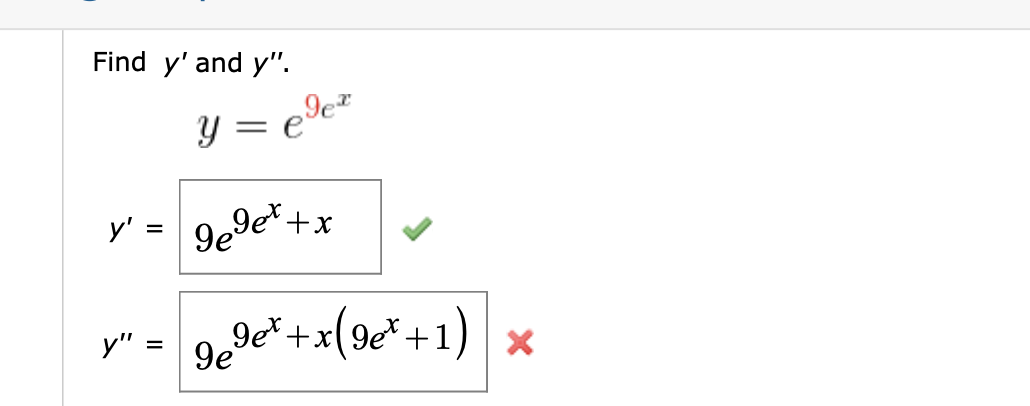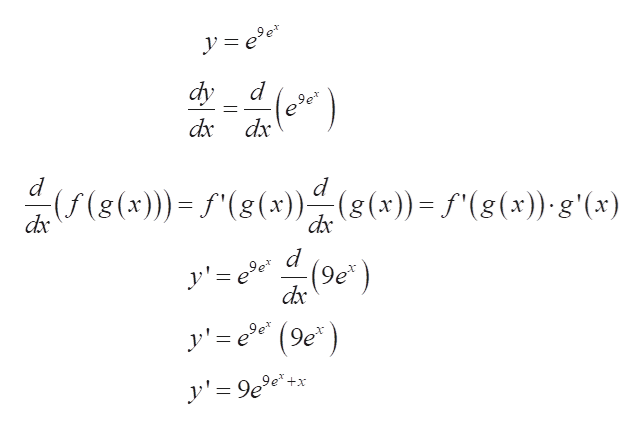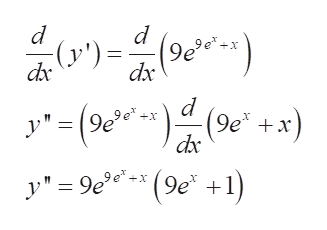Find y'and y".едетy99e*+xу'=+x(e* + 1)9ex9еXу" 3

Questionhelp_outlineImage TranscriptioncloseFind y'and y". едет y 99e*+x у' = +x(e* + 1) 9ex 9е X у" 3 fullscreen
Step 1

Given function is:

Applying derivative with respect to x:

The chain rule of derivative states:

Applying chain rule:help_outlineImage Transcriptionclose= e dy (a) dxc dx (f(g(x)) f(g(x))(g(x))f(g(x)) g'(x) dx dx d y'e(9e y' e(9e +x y'=9e° 11 fullscreen
Step 2

Applying the derivative again and using the chain rule:

(Here, the term in the parent...help_outlineImage Transcriptionclosed d 9e (y') = dx dx d y"=(9e (9e +x) dx y 9e(9e 1 X fullscreen

Want to see the full answer?

See Solution

Want to see this answer and more?

Our solutions are written by experts, many with advanced degrees, and available 24/7

See Solution
Tagged in

Calculus